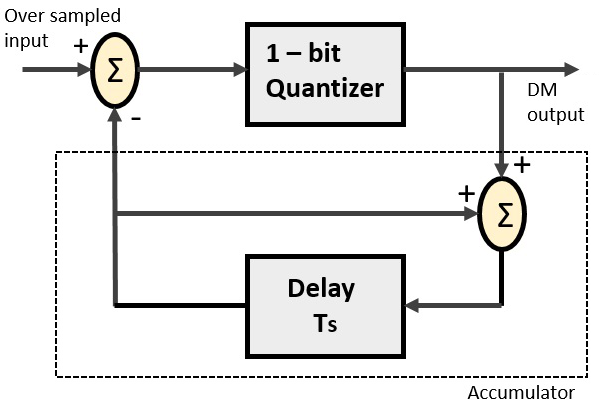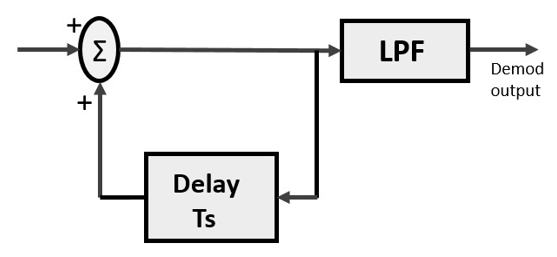# Delta Modulation

The sampling rate of a signal should be higher than the Nyquist rate, to achieve better sampling. If this sampling interval in a Differential PCM (DPCM) is reduced considerably, the sample-to-sample amplitude difference is very small, as if the difference is 1-bit quantization, then the step-size is very small i.e., Δ (delta).

## What is Delta Modulation?

The type of modulation, where the sampling rate is much higher and in which the stepsize after quantization is of smaller value Δ, such a modulation is termed as delta modulation.

### Features of Delta Modulation

• An over-sampled input is taken to make full use of a signal correlation.

• The quantization design is simple.

• The input sequence is much higher than Nyquist rate.

• The quality is moderate.

• The design of the modulator and the demodulator is simple.

• The stair-case approximation of output waveform.

• The step-size is very small, i.e., Δ (delta).

• The bit rate can be decided by the user.

• It requires simpler implementation.

Delta Modulation is a simplified form of DPCM technique, also viewed as 1-bit DPCM scheme. As the sampling interval is reduced, the signal correlation will be higher.

## Delta Modulator

The Delta Modulator comprises of a 1-bit quantizer and a delay circuit along with two summer circuits. Following is the block diagram of a delta modulator.A stair-case approximated waveform will be the output of the delta modulator with the step-size as delta (Δ). The output quality of the waveform is moderate.

## Delta Demodulator

The delta demodulator comprises of a low pass filter, a summer, and a delay circuit. The predictor circuit is eliminated here and hence no assumed input is given to the demodulator.

Following is the block diagram for delta demodulator.Low pass filter is used for many reasons, but the prominent one is noise elimination for out-of-band signals. The step-size error that may occur at the transmitter is called granular noise, which is eliminated here. If there is no noise present, then the modulator output equals the demodulator input.

### Advantages of DM over DPCM

• 1-bit quantizer
• Very easy design of modulator & demodulator

However, there exists some noise in DM and following are the types of noise.

• Slope Over load distortion (when Δ is small)
• Granular noise (when Δ is large)